###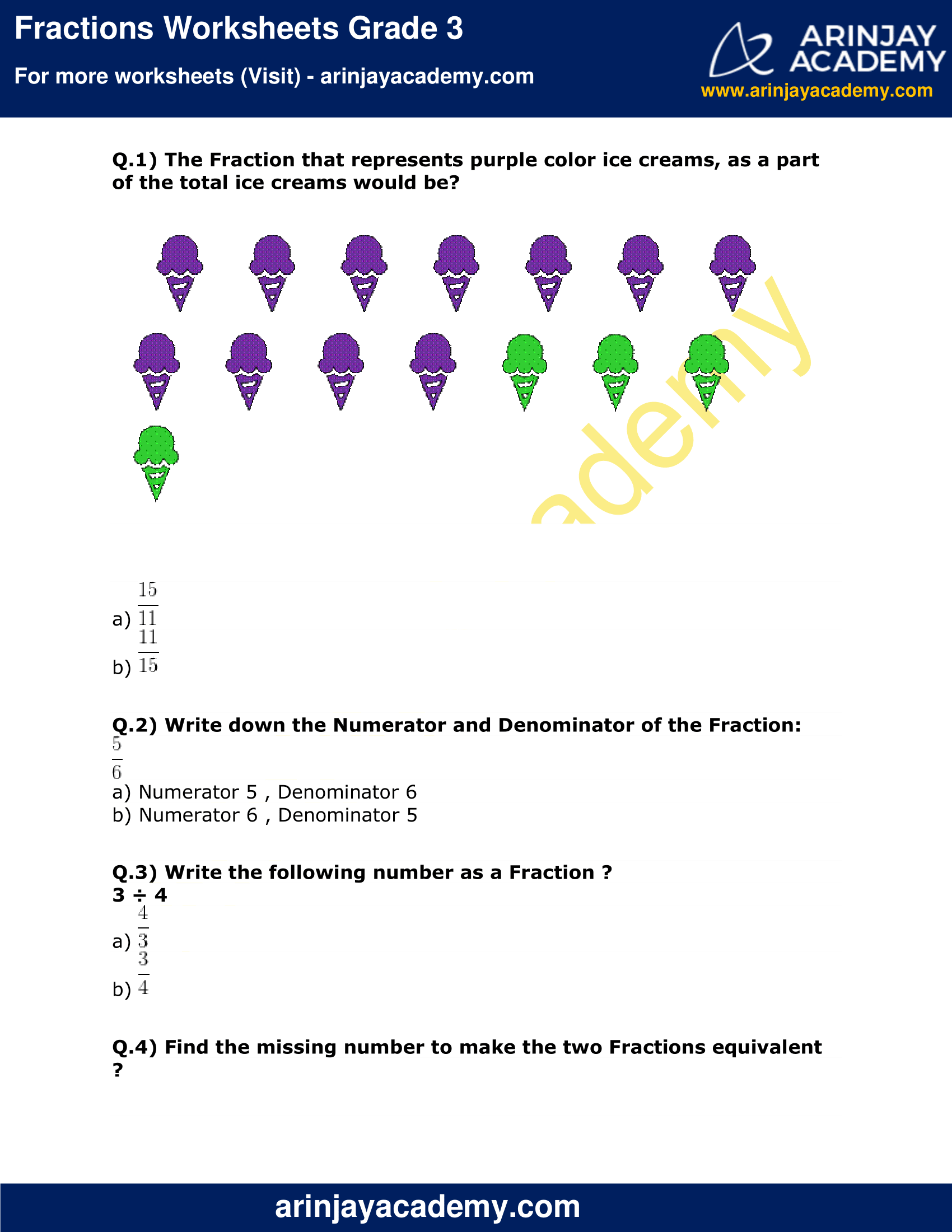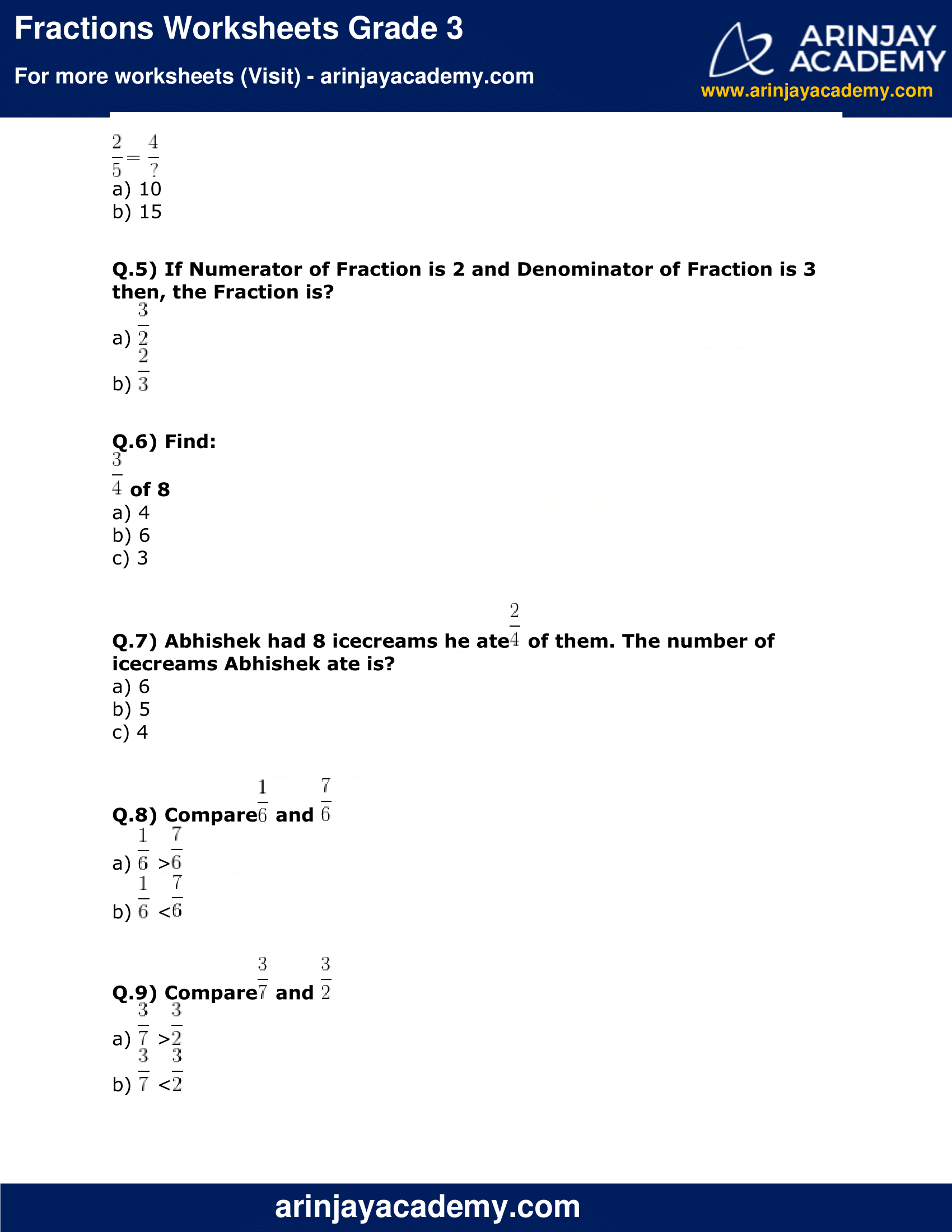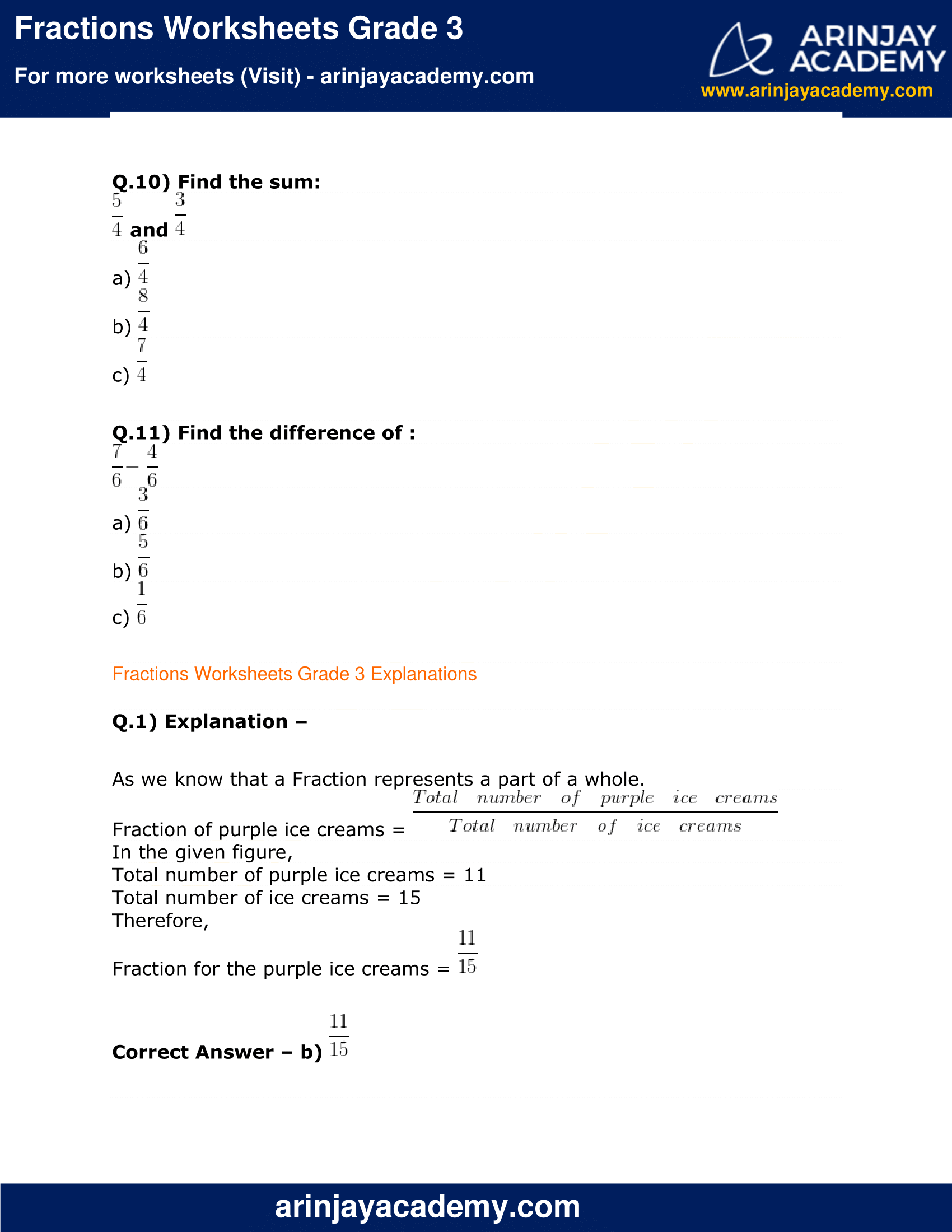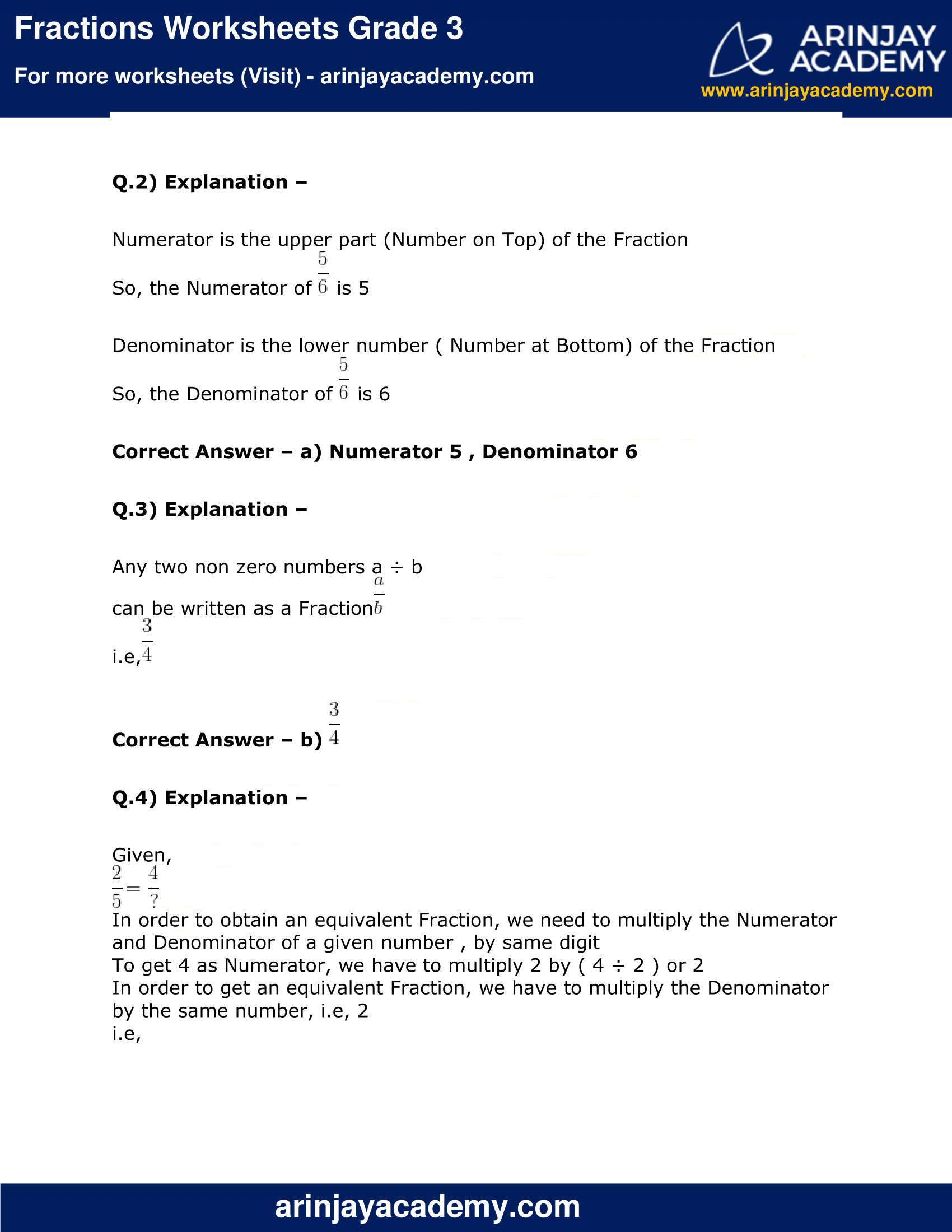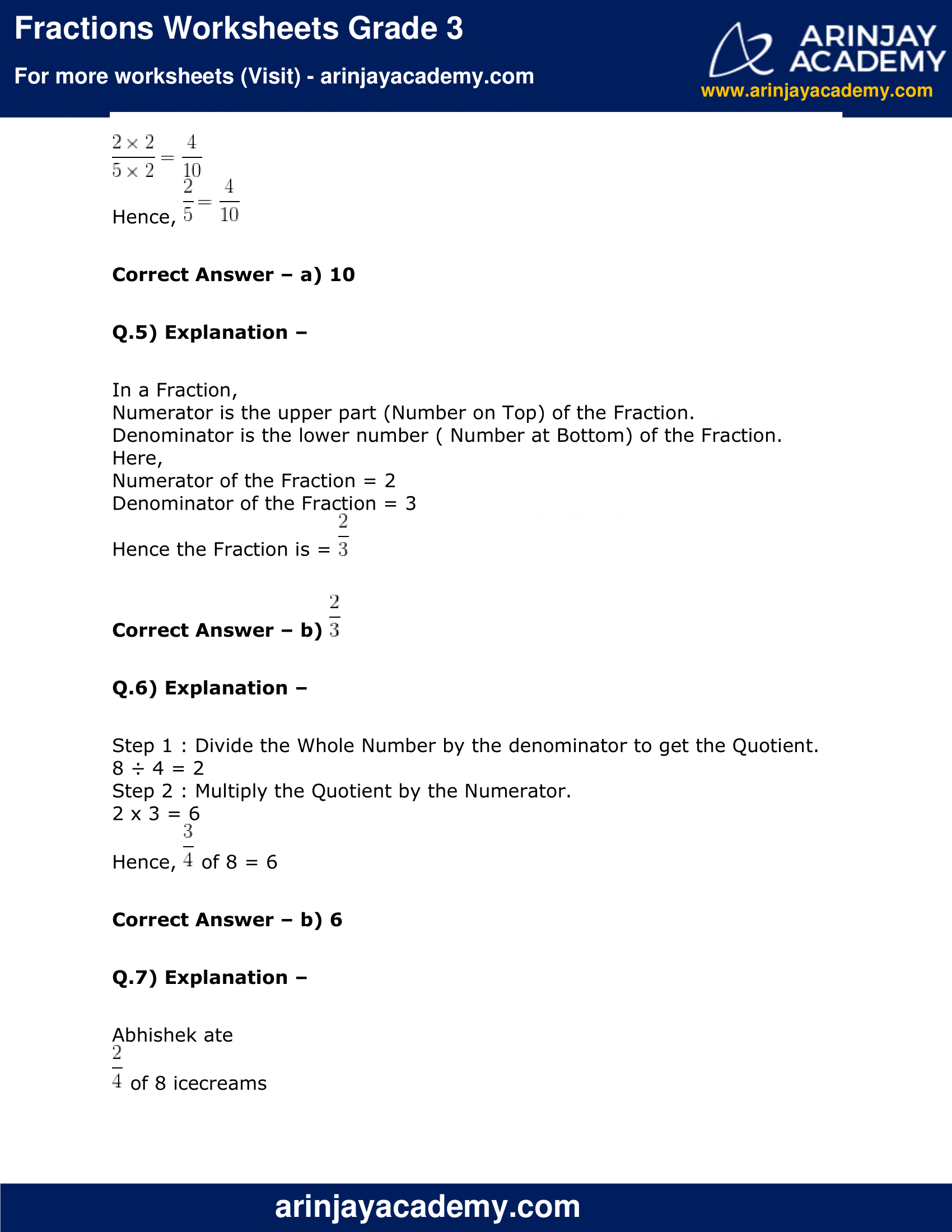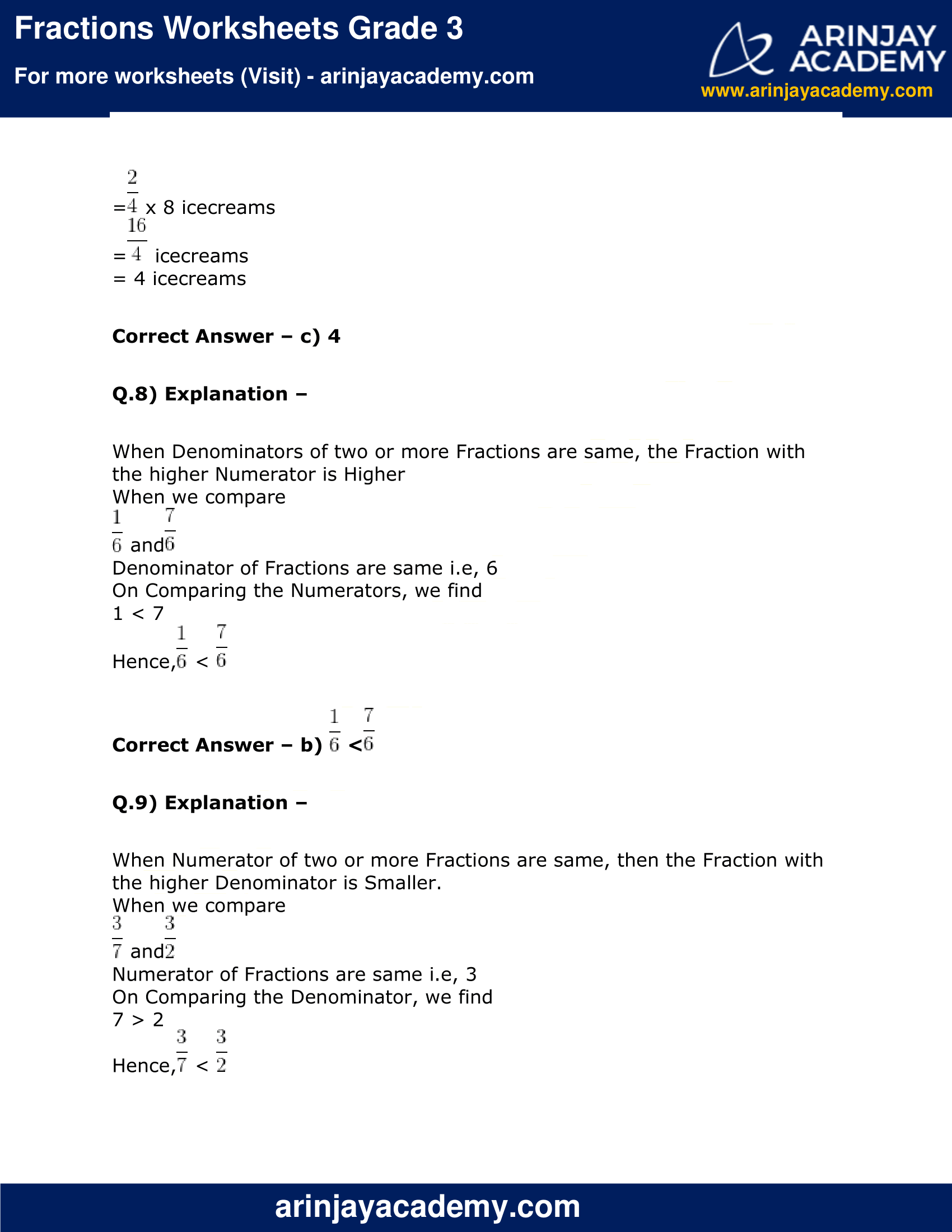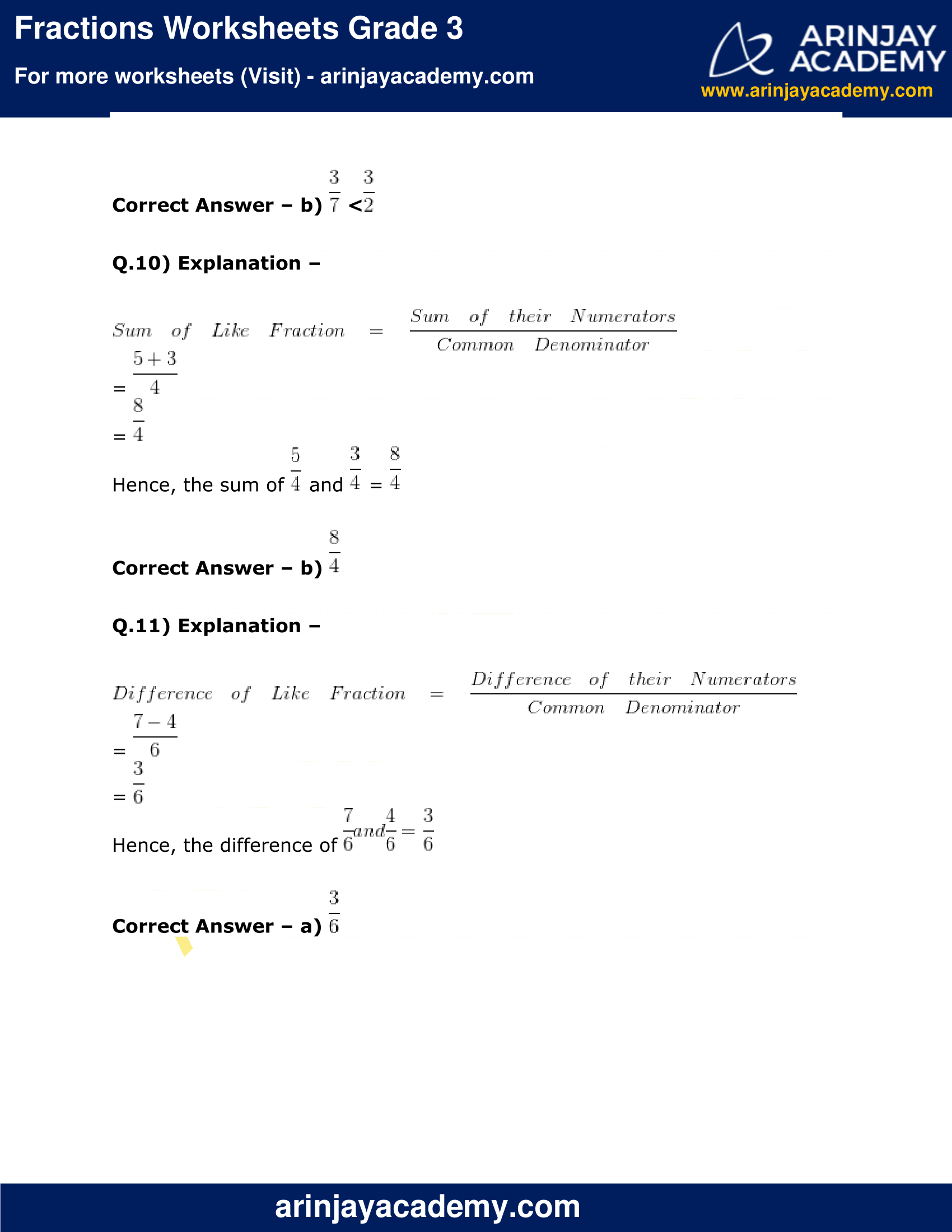Q.1) The Fraction that represents purple color ice creams, as a part of the total ice creams would be?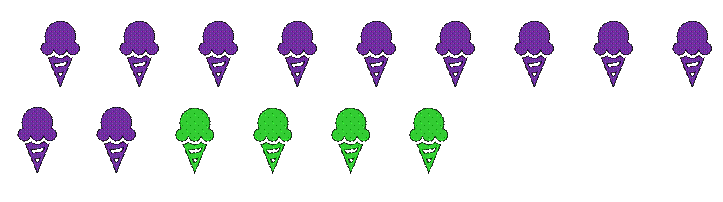a)$\cfrac{15}{11}$
b)$\cfrac{11}{15}$

Q.2) Write down the Numerator and Denominator of the Fraction:$\cfrac{5}{6}$
a) Numerator 5 , Denominator 6
b) Numerator 6 , Denominator 5

Q.3) Write the following number as a Fraction ?
3 ÷ 4
a)$\cfrac{4}{3}$
b)$\cfrac{3}{4}$

Q.4) Find the missing number to make the two Fractions equivalent ?$\cfrac{2}{5} = \cfrac{4}{?}$
a) 10
b) 15

Q.5) If Numerator of Fraction is 2 and Denominator of Fraction is 3 then, the Fraction is?
a)$\cfrac{3}{2}$
b)$\cfrac{2}{3}$

Q.6) Find:$\cfrac{3}{4}$ of 8
a) 4
b) 6
c) 3

Q.7) Abhishek had 8 icecreams he ate$\cfrac { 2 }{ 4 }$ of them. The number of icecreams Abhishek ate is?
a) 6
b) 5
c) 4

Q.8) Compare$\cfrac { 1 }{ 6 }$ and$\cfrac { 7 }{ 6 }$
a)$\cfrac { 1 }{ 6 }$ >$\cfrac { 7 }{ 6 }$
b)$\cfrac { 1 }{ 6 }$ <$\cfrac { 7 }{ 6 }$

Q.9) Compare$\cfrac { 3 }{ 7 }$ and$\cfrac { 3 }{ 2 }$
a)$\cfrac { 3 }{ 7 }$ >$\cfrac { 3 }{ 2 }$
b)$\cfrac { 3 }{ 7 }$ <$\cfrac { 3 }{ 2 }$

Q.10) Find the sum:$\cfrac{5}{4}$ and$\cfrac{3}{4}$
a)$\cfrac{6}{4}$
b)$\cfrac{8}{4}$
c)$\cfrac{7}{4}$

Q.11) Find the difference of :$\cfrac{7}{6} - \cfrac{4}{6}$
a)$\cfrac{3}{6}$
b)$\cfrac{5}{6}$
c)$\cfrac{1}{6}$

### Fractions Worksheets Grade 3 Explanations

Q.1) Explanation –

As we know that a Fraction represents a part of a whole.
Fraction of purple ice creams =$\cfrac { Total\quad number\quad of\quad purple\quad ice\quad creams }{ Total\quad number\quad of\quad ice\quad creams }$
In the given figure,
Total number of purple ice creams = 11
Total number of ice creams = 15
Therefore,
Fraction for the purple ice creams =$\cfrac{11}{15}$

Correct Answer – b)$\cfrac{11}{15}$

Q.2) Explanation –

Numerator is the upper part (Number on Top) of the Fraction
So, the Numerator of$\cfrac{5}{6}$ is 5

Denominator is the lower number ( Number at Bottom) of the Fraction
So, the Denominator of$\cfrac{5}{6}$ is 6

Correct Answer – a) Numerator 5 , Denominator 6

Q.3) Explanation –

Any two non zero numbers a ÷ b
can be written as a Fraction$\cfrac{a}{b}$
i.e,$\cfrac{3}{4}$

Correct Answer – b)$\cfrac{3}{4}$

Q.4) Explanation –

Given,$\cfrac{2}{5} = \cfrac{4}{?}$
In order to obtain an equivalent Fraction, we need to multiply the Numerator and Denominator of a given number , by same digit
To get 4 as Numerator, we have to multiply 2 by ( 4 ÷ 2 ) or 2
In order to get an equivalent Fraction, we have to multiply the Denominator by the same number, i.e, 2
i.e,$\cfrac { 2\times 2 }{5\times 2} = \cfrac{4}{10}$
Hence,$\cfrac{2}{5} = \cfrac{4}{10}$

Q.5) Explanation –

In a Fraction,
Numerator is the upper part (Number on Top) of the Fraction.
Denominator is the lower number ( Number at Bottom) of the Fraction.
Here,
Numerator of the Fraction = 2
Denominator of the Fraction = 3
Hence the Fraction is =$\cfrac{2}{3}$

Correct Answer – b)$\cfrac{2}{3}$

Q.6) Explanation –

Step 1 : Divide the Whole Number by the denominator to get the Quotient.
8 ÷ 4 = 2
Step 2 : Multiply the Quotient by the Numerator.
2 x 3 = 6
Hence,$\cfrac{3}{4}$ of 8 = 6

Q.7) Explanation –

Abhishek ate$\cfrac { 2 }{ 4 }$ of 8 icecreams
=$\cfrac { 2 }{ 4 }$ x 8 icecreams
=$\cfrac { 16 }{ 4 }$ icecreams
= 4 icecreams

Q.8) Explanation –

When Denominators of two or more Fractions are same, the Fraction with the higher Numerator is Higher
When we compare$\cfrac { 1 }{ 6 }$ and$\cfrac { 7 }{ 6 }$
Denominator of Fractions are same i.e, 6
On Comparing the Numerators, we find
1 < 7
Hence,$\cfrac { 1 }{ 6 }$ <$\cfrac { 7 }{ 6 }$

Correct Answer – b)$\cfrac { 1 }{ 6 }$ <$\cfrac { 7 }{ 6 }$

Q.9) Explanation –

When Numerator of two or more Fractions are same, then the Fraction with the higher Denominator is Smaller.
When we compare$\cfrac { 3 }{ 7 }$ and$\cfrac { 3 }{ 2 }$
Numerator of Fractions are same i.e, 3
On Comparing the Denominator, we find
7 > 2
Hence,$\cfrac { 3 }{ 7 }$ <$\cfrac { 3 }{ 2 }$

Correct Answer – b)$\cfrac { 3 }{ 7 }$ <$\cfrac { 3 }{ 2 }$

Q.10) Explanation –$Sum\quad of\quad Like\quad Fraction\quad =\quad \cfrac { Sum\quad of\quad their\quad Numerators }{ Common\quad Denominator }$
=$\cfrac{5+3}{4}$
=$\cfrac{8}{4}$
Hence, the sum of$\cfrac{5}{4}$ and$\cfrac{3}{4}$ =$\cfrac{8}{4}$

Correct Answer – b)$\cfrac{8}{4}$

Q.11) Explanation –$Difference\quad of\quad Like\quad Fraction\quad =\quad \cfrac { Difference\quad of\quad their\quad Numerators }{ Common\quad Denominator }$
=$\cfrac{7 - 4}{6}$
=$\cfrac{3}{6}$
Hence, the difference of$\cfrac{7}{6} and \cfrac{4}{6} = \cfrac{3}{6}$

Correct Answer – a)$\cfrac{3}{6}$